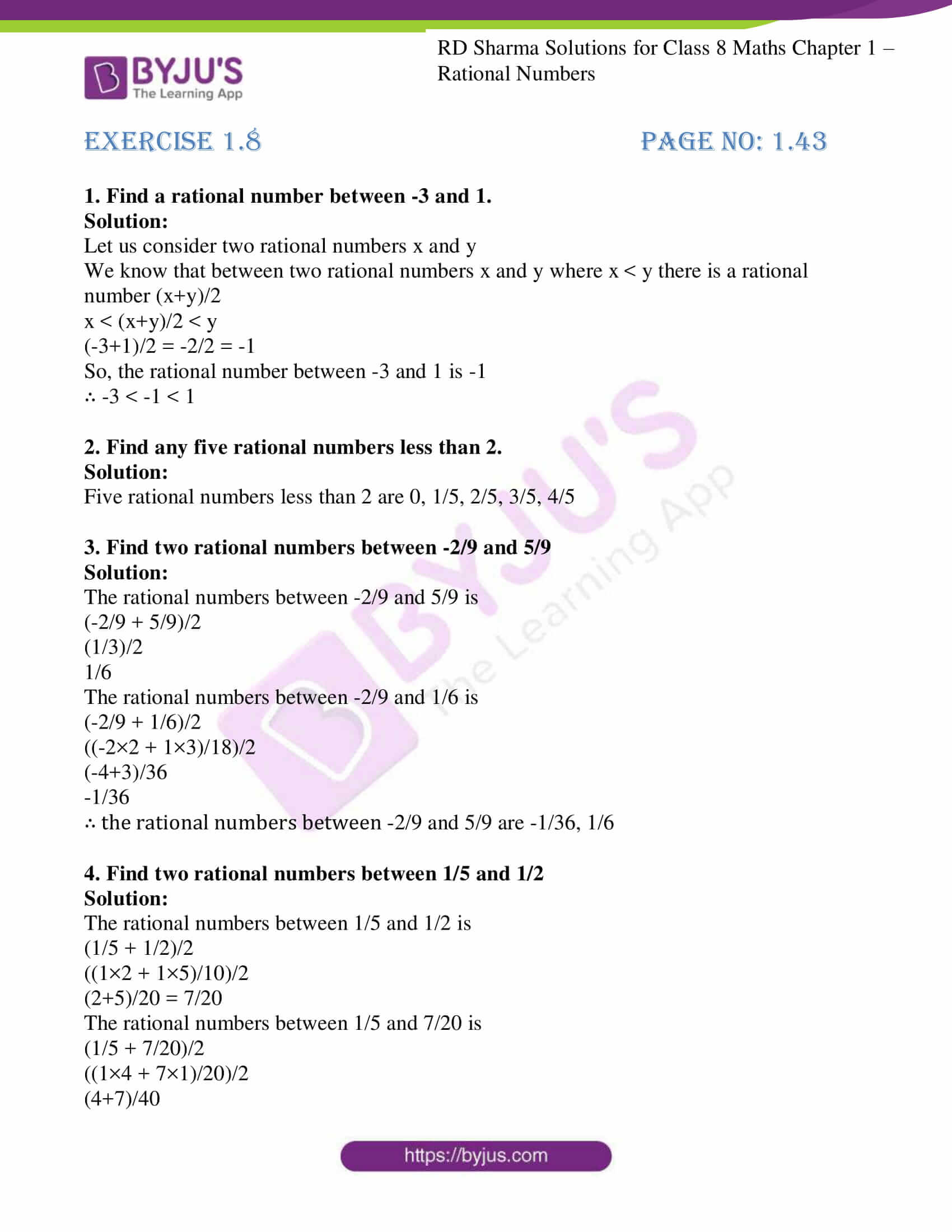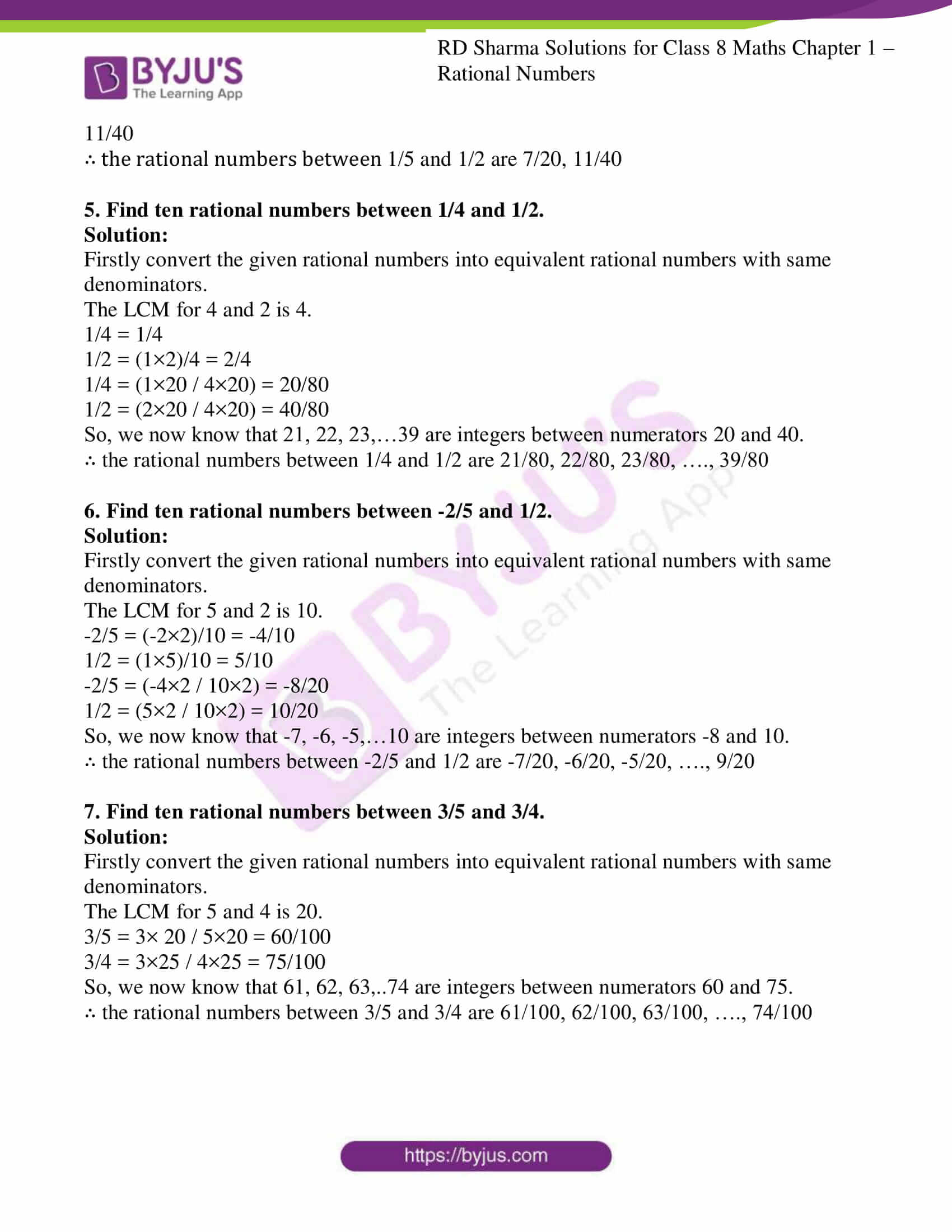# RD Sharma Solutions for Class 8 Maths Chapter 1 - Rational Numbers Exercise 1.8

RD Sharma Solutions for Class 8 Maths Exercise 1.8 Chapter 1, Rational Numbers. Students can download from the links provided below. This set of solutions are designed by our expert team in order to help students excel in their exam with flying colours. In Exercise 1.8 of RD Sharma Class 8 Solution for Maths, we shall discuss problems based on the representation of rational numbers on the number line, rational numbers between two rational numbers.

## Download PDF of RD Sharma Solutions for Class 8 Maths Exercise 1.8 Chapter 1 Rational Numbers### Access Answers to RD Sharma Solutions for Class 8 Maths Exercise 1.8 Chapter 1 Rational Numbers

1. Find a rational number between -3 and 1.

Solution:

Let us consider two rational numbers x and y

We know that between two rational numbers x and y where x < y there is a rational number (x+y)/2

x < (x+y)/2 < y

(-3+1)/2 = -2/2 = -1

So, the rational number between -3 and 1 is -1

∴ -3 < -1 < 1

2. Find any five rational numbers less than 2.

Solution:

Five rational numbers less than 2 are 0, 1/5, 2/5, 3/5, 4/5

3. Find two rational numbers between -2/9 and 5/9

Solution:

The rational numbers between -2/9 and 5/9 is

(-2/9 + 5/9)/2

(1/3)/2

1/6

The rational numbers between -2/9 and 1/6 is

(-2/9 + 1/6)/2

((-2×2 + 1×3)/18)/2

(-4+3)/36

-1/36

∴ the rational numbers between -2/9 and 5/9 are -1/36, 1/6

4. Find two rational numbers between 1/5 and 1/2

Solution:

The rational numbers between 1/5 and 1/2 is

(1/5 + 1/2)/2

((1×2 + 1×5)/10)/2

(2+5)/20 = 7/20

The rational numbers between 1/5 and 7/20 is

(1/5 + 7/20)/2

((1×4 + 7×1)/20)/2

(4+7)/40

11/40

∴ the rational numbers between 1/5 and 1/2 are 7/20, 11/40

5. Find ten rational numbers between 1/4 and 1/2.

Solution:

Firstly convert the given rational numbers into equivalent rational numbers with same denominators.

The LCM for 4 and 2 is 4.

1/4 = 1/4

1/2 = (1×2)/4 = 2/4

1/4 = (1×20 / 4×20) = 20/80

1/2 = (2×20 / 4×20) = 40/80

So, we now know that 21, 22, 23,…39 are integers between numerators 20 and 40.

∴ the rational numbers between 1/4 and 1/2 are 21/80, 22/80, 23/80, …., 39/80

6. Find ten rational numbers between -2/5 and 1/2.

Solution:

Firstly convert the given rational numbers into equivalent rational numbers with same denominators.

The LCM for 5 and 2 is 10.

-2/5 = (-2×2)/10 = -4/10

1/2 = (1×5)/10 = 5/10

-2/5 = (-4×2 / 10×2) = -8/20

1/2 = (5×2 / 10×2) = 10/20

So, we now know that -7, -6, -5,…10 are integers between numerators -8 and 10.

∴ the rational numbers between -2/5 and 1/2 are -7/20, -6/20, -5/20, …., 9/20

7. Find ten rational numbers between 3/5 and 3/4.

Solution:

Firstly convert the given rational numbers into equivalent rational numbers with same denominators.

The LCM for 5 and 4 is 20.

3/5 = 3× 20 / 5×20 = 60/100

3/4 = 3×25 / 4×25 = 75/100

So, we now know that 61, 62, 63,..74 are integers between numerators 60 and 75.

∴ the rational numbers between 3/5 and 3/4 are 61/100, 62/100, 63/100, …., 74/100

## RD Sharma Solutions for Class 8 Maths Exercise 1.8 Chapter 1 Rational Numbers

Class 8 Maths Chapter 1 Rational Numbers Exercise 1.8 is based on the representation of rational numbers on the number line. To facilitate easy learning and understanding of concepts download free RD Sharma Solutions Chapter 1 which provides answers to all the questions. Practising as many times as possible helps students obtain high marks.